#### Solving a query

Once the roadmap is obtained, it is straightforward to solve a motion planning query,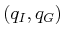. Let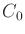and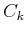denote the cells that contain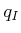and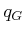, respectively. In the graph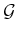, search for a path that connects the sample point ofto the sample point of. If no such path exists, then the planning algorithm correctly declares that no solution exists. If one does exist, then let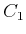,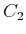,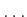,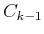denote the sequence of 1-cells and 2-cells visited along the computed path infromto.

A solution path can be formed by simply connecting the dots.'' Let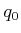,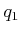,,,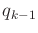,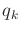, denote the sample points along the path in. There is one sample point for every cell that is crossed. The solution path,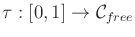, is formed by setting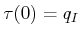,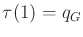, and visiting each of the points in the sequence fromtoby traveling along the shortest path. For the example, this leads to the solution shown in Figure 6.5. In selecting the sample points, it was important to ensure that each path segment from the sample point of one cell to the sample point of its neighboring cell is collision-free.6.4

Steven M LaValle 2020-08-14